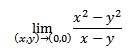# How to prove that a limit of a two-variable function does not exist?I need to prove this limit does NOT exist. I know that the denominator cancels out, however my lecturer says the limit does not exist because we cannot find a circle with radius $\delta$ that contains the point (0,0), as the function is not defined for all y=x. Here is a little drawing of the domain.

Is my lecturer's explanation valid?

Thanks in advance,
Michael

## Answers and Replies

Rationalize it

I guess his explanation makes sense, but that's a funny way of putting it.

I guess his explanation makes sense, but that's a funny way of putting it.

Can you think of another way? If you do, please post

SammyS
Staff Emeritus
Science Advisor
Homework Helper
Gold MemberI need to prove this limit does NOT exist. I know that the denominator cancels out, however my lecturer says the limit does not exist because we cannot find a circle with radius $\delta$ that contains the point (0,0), as the function is not defined for all y=x. Here is a little drawing of the domain.

Is my lecturer's explanation valid?

Thanks in advance,
Michael
Well, that limit certainly does exist --- at least it exists using the mufti-variable definition of limit with which I am familiar.

$\displaystyle \text{If }x\ne y\,,\text{ then }\frac{x^2-y^2}{x-y}=\frac{(x-y)(x+y)}{x-y}=x+y\,.$ No matter what continuous path you take (in the domain of the given function) to approach (0,0), x+y → 0 .

On the other hand, the function is not continuous at (x,y) = (0,0) because it's not defined at (x,y) = (0,0) .

LCKurtz
Science Advisor
Homework Helper
Gold Member
I think it is common for functions of two variables to be required to be defined in a punctured disk around (a,b) in order to claim a limit as ##(x,y)\to (a,b)## of the function exist. That is presumably required in this case, given the lecturer's statement.

Well, that limit certainly does exist --- at least it exists using the mufti-variable definition of limit with which I am familiar.

$\displaystyle \text{If }x\ne y\,,\text{ then }\frac{x^2-y^2}{x-y}=\frac{(x-y)(x+y)}{x-y}=x+y\,.$ No matter what continuous path you take (in the domain of the given function) to approach (0,0), x+y → 0 .

On the other hand, the function is not continuous at (x,y) = (0,0) because it's not defined at (x,y) = (0,0) .

Well, as far as I know, the limit does not have to be defined at the point itself (0,0), but there must exist at least one disc that contains it.

LCKurtz
Science Advisor
Homework Helper
Gold Member
Well, as far as I know, the limit does not have to be defined at the point itself (0,0), but there must exist at least one disc that contains it.

Yes, there must be a punctured disk around (a,b) in the domain of the function, which you don't have.

Yes, there must be a punctured disk around (a,b) in the domain of the function, which you don't have.

Alright, thanks a lot!

HallsofIvy
Science Advisor
Homework Helper
Well, that limit certainly does exist --- at least it exists using the mufti-variable definition of limit with which I am familiar.

$\displaystyle \text{If }x\ne y\,,\text{ then }\frac{x^2-y^2}{x-y}=\frac{(x-y)(x+y)}{x-y}=x+y\,.$ No matter what continuous path you take (in the domain of the given function) to approach (0,0), x+y → 0 .
Even the path y= x??

On the other hand, the function is not continuous at (x,y) = (0,0) because it's not defined at (x,y) = (0,0) .

SammyS
Staff Emeritus
Science Advisor
Homework Helper
Gold Member
Even the path y= x??
y=x is not in the domain of $\displaystyle \frac{x^2-y^2}{x-y}\ .$

It appears that Calculus textbooks don't agree on the definition of the limit for functions of more than one variable.